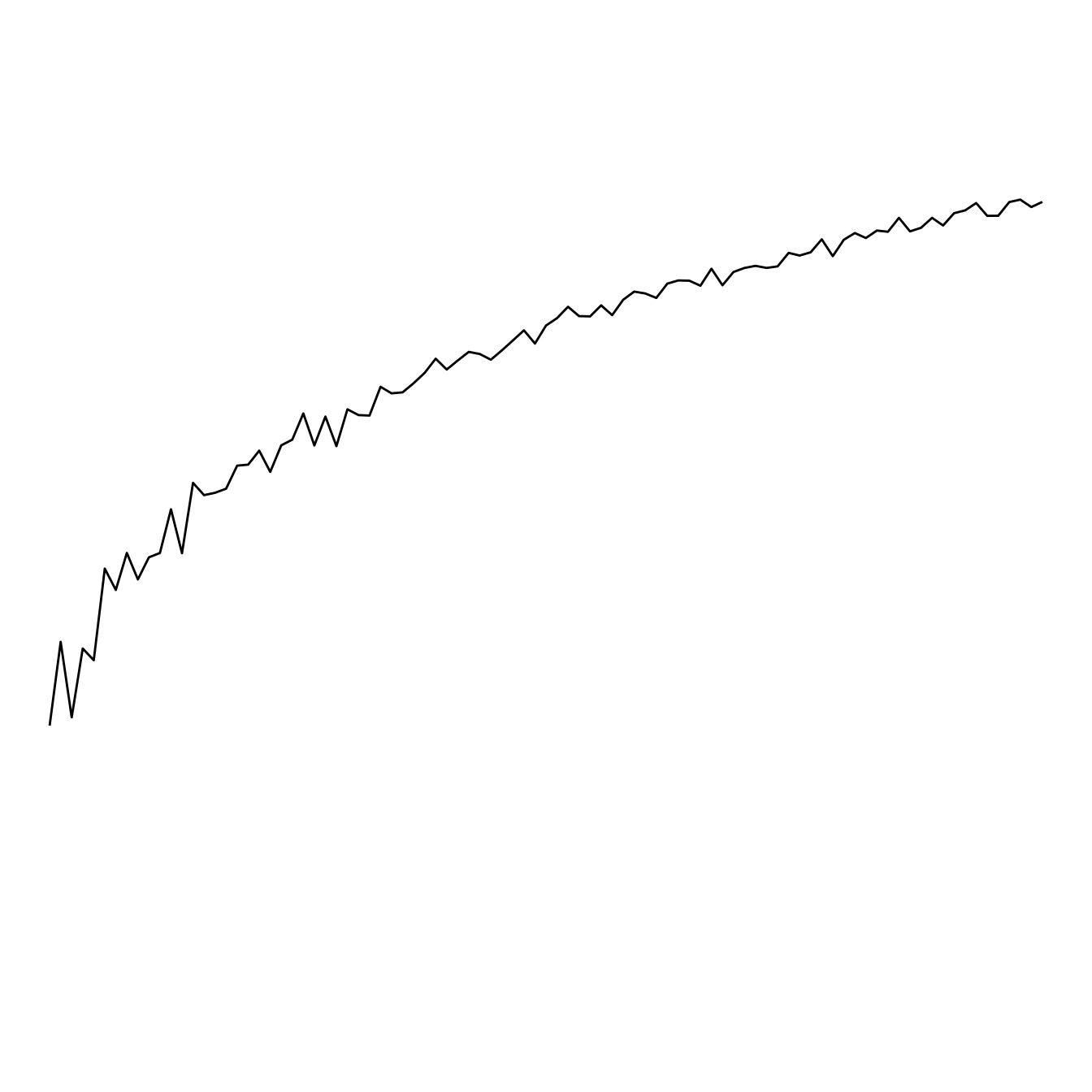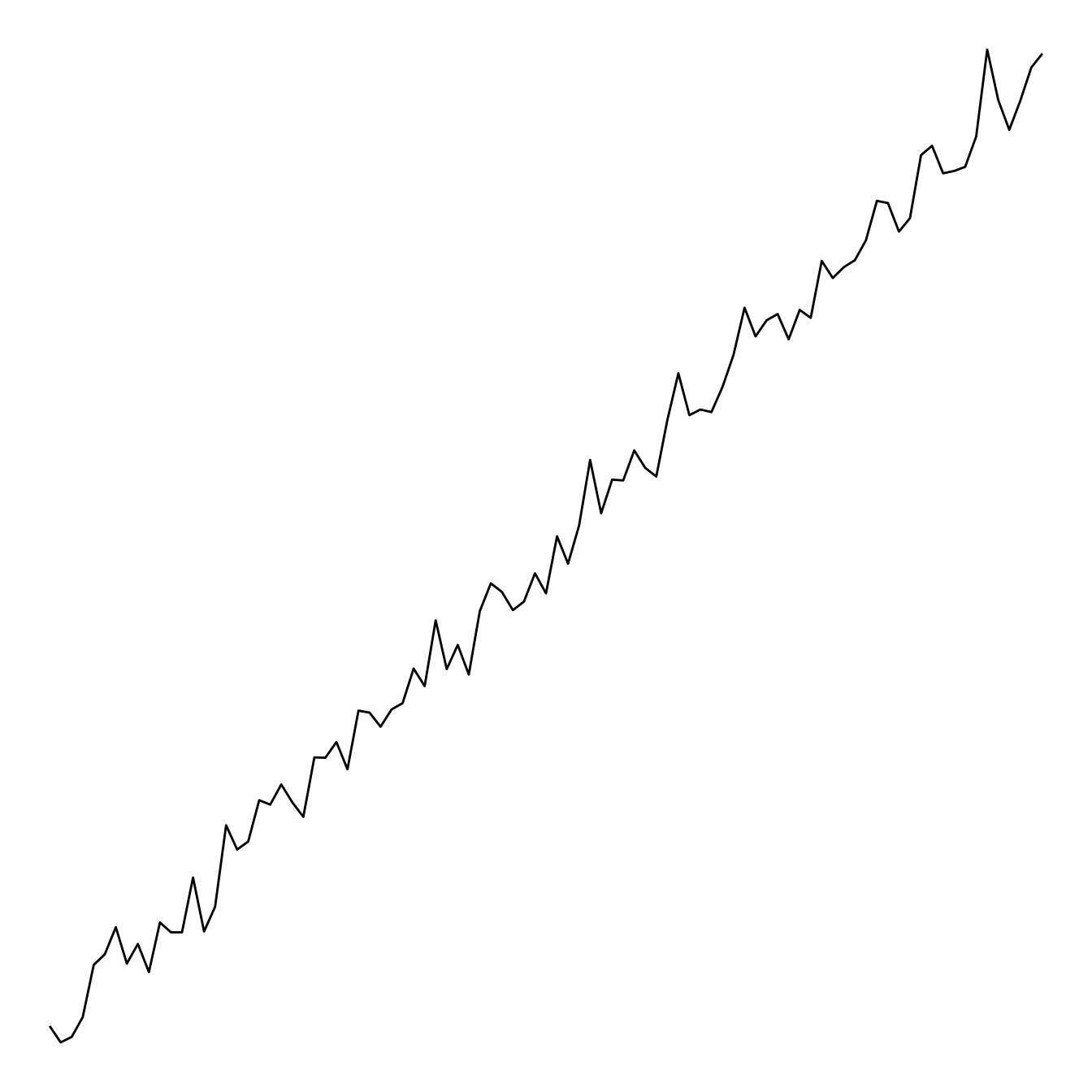# Line plot with log scale

This post explaines how to build a line chart with a log scale for its Y axis, using the `scale_y_log10` function.

It is sometimes useful to use a log scale for a numeric variable. Indeed, it allows to “magnify” the lower part of the curve.

This is possible thanks to the `scale_y_log10()` function. Control the horizontal grid lines with `breaks`, and the axis limits with `limits`. (Note that 0 is not allowed, since log(0) is not defined.)``````# Library
library(ggplot2)

# Create dummy data
data <- data.frame(
x=seq(10,100),
y=seq(10,100)/2+rnorm(90)
)

# Make the plot
ggplot(data, aes(x=x, y=y)) +
geom_line() +
scale_y_log10( breaks=c(1,5,10,15,20,50,100), limits=c(1,100) )``````

# Without log transform

This is the same chart without the log transform:``````# Library
library(ggplot2)

# Create dummy data
data <- data.frame(
x=seq(10,100),
y=seq(10,100)/2+rnorm(90)
)

# Make the plot
ggplot(data, aes(x=x, y=y)) +
geom_line() ``````

Related chart types

## Contact

This document is a work by Yan Holtz. Any feedback is highly encouraged. You can fill an issue on Github, drop me a message on Twitter, or send an email pasting yan.holtz.data with gmail.com.